## 前言

Logistic 回归和 Softmax 回归解决的是线性分类问题，即不同类别之间可以被线性平面分隔开，所以相当于没有隐藏层的神经网络。对于线性不可分的数据，由于线性模型无法理解任何两个特征间的相互作用，所以就需要有隐藏层(使用了非线性激活函数)的神经网络提取特征，将线性不可分的数据变得线性可分。

## 单隐层神经网络

### 特征提取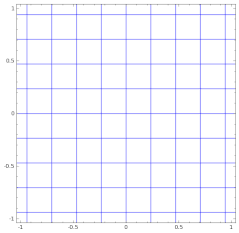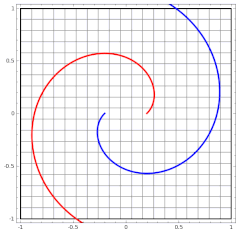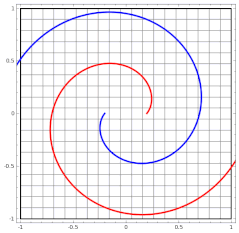## 单隐层分类平面数据

### 数据集### 神经网络模型

• Tanh 函数是双曲正切函数$$tanh(x)=\frac{sinhx}{coshx}=\frac{e^x-e^{-x}}{e^x+e^{-x}}$$

$$tanh’(x)=sech^2x=1-tanh^2x$$

Tanh 和 Sigmoid 函数可以通过缩放平移重合，为什么 Tanh 函数表现更好？Deep Learning 中给出的解释是：因为 Tanh 函数经过原点，且在原点附近梯度比 Sigmoid 函数的梯度大，所以在训练过程中优化会比较容易。

Tanh 和 Sigmoid 函数能否拟合任意函数呢？不能！首先来看一下什么叫 Squashing (压扁；压制)函数：

A function $\Psi: R\to[0, 1]$ is a squashing function if it is non-decreasing, $\lim\limits_{\lambda \to \infty }{\Psi(\lambda)}=1$ and $\lim\limits_{\lambda \to -\infty }{\Psi(\lambda)}=0$.

显然 Tanh 函数通过缩放平移和 Sigmoid 函数满足这个定义，那么 Squashing 函数有什么性质呢？Hornik 等人在 1989 年中的一篇文章中说道：即使单隐层神经网络，用任意的 Squashing 函数作为激活函数，当神经元数量足够多时，可以拟合任意的博雷尔可测(Borel measurable)函数。那么博雷尔不可测函数又是什么意思呢？

定义集合 $S$ 为一个博雷尔不可测集合，有
$$f(x) = \begin{cases} 0 & x \not\in S \\ 1 & x \in S \end{cases}$$
则函数 $f(x)$ 就是博雷尔不可测函数。那么博雷尔不可测集合又是什么呢？这就触及到我的知识盲区了，总之用 Squashing 函数拟合实际问题中的函数是绰绰有余的，也不难想象足够多的 Squashing 函数的线性组合确实能拟合很多函数了。

• 模型结构：$$z^{ (i)} = W^{} x^{(i)} + b^{}\tag{1}$$

$$a^{ (i)} = \tanh(z^{ (i)})\tag{2}$$

$$z^{ (i)} = W^{} a^{ (i)} + b^{}\tag{3}$$

$$\hat{y}^{(i)} = a^{ (i)} = \sigma(z^{  (i)})\tag{4}$$

$$y^{(i)}_{prediction} = \begin{cases} 1 & \mbox{if}\quad a^{(i)} > 0.5 \\ 0 & \mbox{otherwise} \end{cases}\tag{5}$$

$$J = - \frac{1}{m} \sum\limits_{i = 0}^{m} \left(y^{(i)}\log(a^{ (i)}) + (1-y^{(i)})\log(1- a^{ (i)})\right)\tag{6}$$

1. 定义神经网络结构(神经元的个数、隐藏层的层数等)
2. 初始化模型参数
3. 循环
• 实现前向传播(实现公式 1~4，得到预测值 $\hat y$，即 $a^{}$)
• 计算代价(根据前向传播得到的预测值和测试集的标签，实现公式 6，得到代价)
• 实现反向传播，计算梯度
• 梯度下降更新模型参数

#### 初始化模型参数

• 如果权值全部初始化为相同的数，那么隐藏层中神经元的输出就都是一样的，通过归纳法可以归纳出这些隐藏层的神经元一直在计算完全一样的函数，所以需要随机初始化打破对称性；
• 如果权值全部初始化为 0，更加糟糕的是不管输入是什么，隐藏层中神经元的输出就都是 0；
• 如果初始化为比较大的数，那么就会导致激活函数输出的值比较大，梯度较小，梯度下降的速度较慢，所以需要初始化为 0 附近的随机数。

#### 循环

##### 反向传播

• 对于一个样本数据 $x$ (随机梯度下降)，有：

$$\mathscr{l}(\hat y, y)=-\left(y^{(i)}\log(a^{ (i)}) + (1-y^{(i)})\log(1- a^{ (i)})\right)$$

\begin{align} dz^{}=\frac{\partial{\mathscr{l}(\hat y, y)}}{\partial{z^{}}} & = -\left(\frac{y}{a^{}}\sigma’(z^{})+\frac{1-y^{(i)}}{1-a^{}}\sigma’(z^{})\right) \\ & = a^{}-y \end{align}

\begin{align} dW^{}=\frac{\partial{\mathscr{l}(\hat y, y)}}{\partial{z^{}}}\frac{\partial{z^{}}}{\partial{W^{}}} & = dz^{}a^{\mathrm{T}} \end{align}

$$db^{}=\frac{\partial{\mathscr{l}(\hat y, y)}}{\partial{z^{}}}\frac{\partial{z^{}}}{\partial{b^{}}}=dz^{}$$

\begin{align} dz^{} = W^{\mathrm{T}}dz^{}*\left(1-tanh^2(z)\right) = W^{\mathrm{T}}dz^{}*(1-a^{2}) \end{align}

$$dW^{}=dz^{}x^{\mathrm{T}}$$

$$db^{}=dz^{}$$

$$dz^{}=da^{}*\frac{\partial{a^{}}}{\partial{z^{}}}=W^{\mathrm{T}}dz^{}*(1-a^{2})$$
$m$ 表示样本数量($m = 1$)，由于 $a^{}$ 和 $z^{}$ 只是进行了一个非线性变换，具有相同的维度，所以用点乘；所以只需要求 $da^{}$ 且满足 $da^{}.shape=(n^{[h]}, m)$：
$$(n^{[h]}, m) = (n^{[y]}, n^{[h]})^{\mathrm{T}}(n^{[y]}, m) = (n^{[h]}, n^{[y]})(n^{[y]}, m)$$

• 对于所有样本数据，有：

$$dZ^{}=A^{}-Y$$

$$dW^{}=\frac{1}{m}dZ^{}A^{\mathrm{T}}$$

$$db^{}=\frac{1}{m}\sum\limits_{i = 0}^{m}dZ^{}$$

$$dZ^{}=W^{\mathrm{T}}dZ^{}*(1-A^{2})$$
$$dW^{}=\frac{1}{m}dZ^{}X^{\mathrm{T}}$$

$$db^{}=\frac{1}{m}\sum\limits_{i = 0}^{m}dZ^{}$$

##### 梯度下降更新模型参数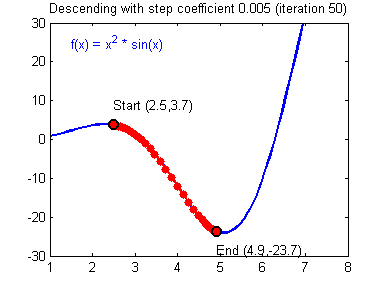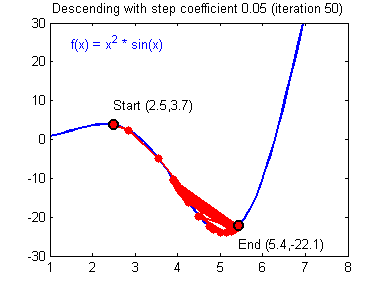### 评估分析## 参考文献

 吴恩达. DeepLearning.

 Ian Goodfellow, Yoshua Bengio, Aaron Courville. Deep Learning. 人民邮电出版社. 2017.

 Hornik, K., Stinchcombe, M., & White, H. (1989). Multilayer feedforward networks are universal approximators. Neural networks, 2(5), 359-366.

0%